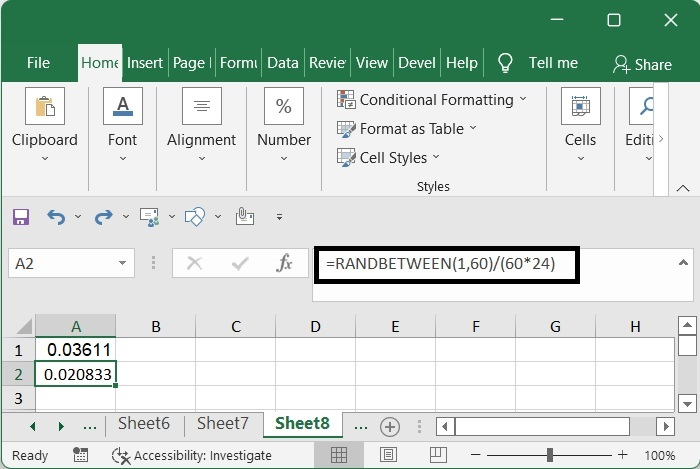# Excel random data: generate random numbers, texts, dates, times in Excel

When faced with a frequent scenario, the majority of us may be required to input arbitrary numbers, dates, or text strings according to our preferences. However, at times, have you ever attempted to insert times into a range of cells in a random range?

This function's name is RAND(). Excel will return a number that is either higher than or equal to 0 (zero), but it will never return 1. It is possible to generate random integers, text, date and time values, and even dates and times by combining the RAND() function with a few additional functions.

## Random numbers

shows how the RAND function generates a value that is either more than or equal to 0 (zero), but is less than 1, whenever a worksheet is updated.

If you press the F9 key, all of the sheets in all of the active workbooks will be recalculated, and cell A1 will get a new random number as a result.

=RAND()


Choose a cell that is blank, such as A1, and then use the formula from above.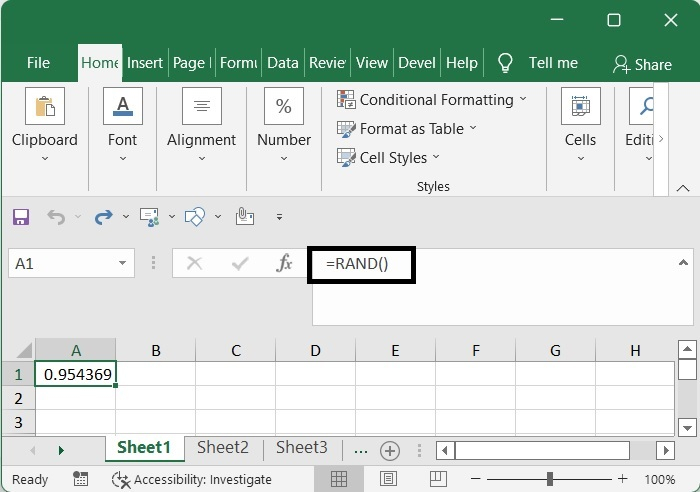You will get a random number ranging from 0 to 1 if you use this formula.

## Return values that are either between or equal to

This function will return values that are less than or equal to 0 and more than 10.

### ROUNDUP()

=ROUNDUP(RAND()*11, 0)-1


Using ROUNDUP () get random number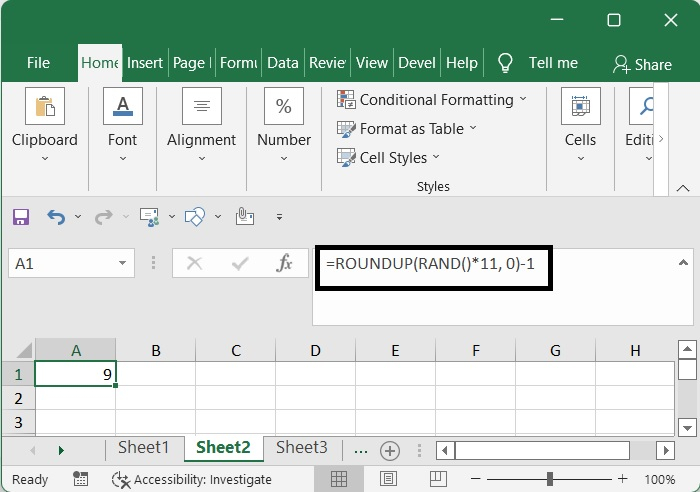### ANDBETWEEN()

Using ANDBETWEEN() get random number

=RANDBETWEEN(1, 10)


Using RANDBETWEEN () get random number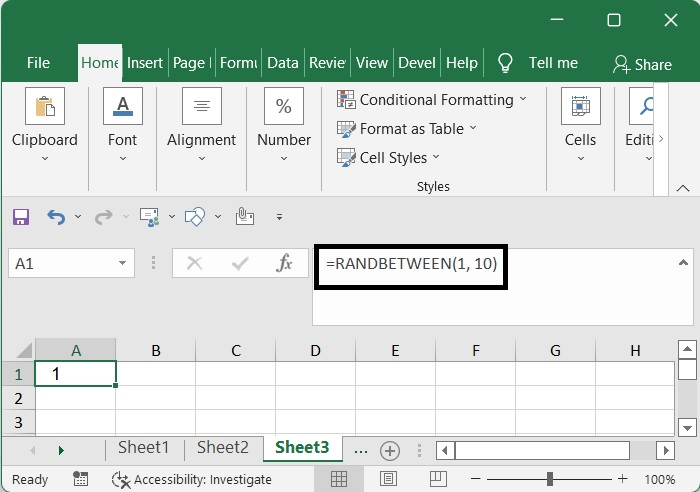If you use this formula, you will be able to generate a random number between 0 and 10, and you may customize it.

## Random texts

Excel assigns a number to each character, and that number is determined by the character set that is being used (ANSI on Windows). The numbers from A to Z range from 65 to 90, and the numbers from 97 to 122. Excel assigns a number to each character, and that number is determined by the character set that is being used (ANSI on Windows). The numbers from A to Z range from 65 to 90, and the numbers from 97 to 122.

returns the character from the character set of your machine that corresponds to the code number that you provided bellow.

=CHAR(number)


It is possible to return random characters by using the CHAR, RAND, and ROUNDUP functions; let me now demonstrate how to do so.

### four random uppercase letters

By using the formula shown below, you should get four random uppercase letters.

=CHAR(ROUNDUP(RAND()*26, 0)-1+65)&CHAR(ROUNDUP(RAND()*26, 0)-1+65)&CHAR(ROUNDUP(RAND()*26, 0)-1+65)&CHAR(ROUNDUP(RAND()*26, 0)-1+65)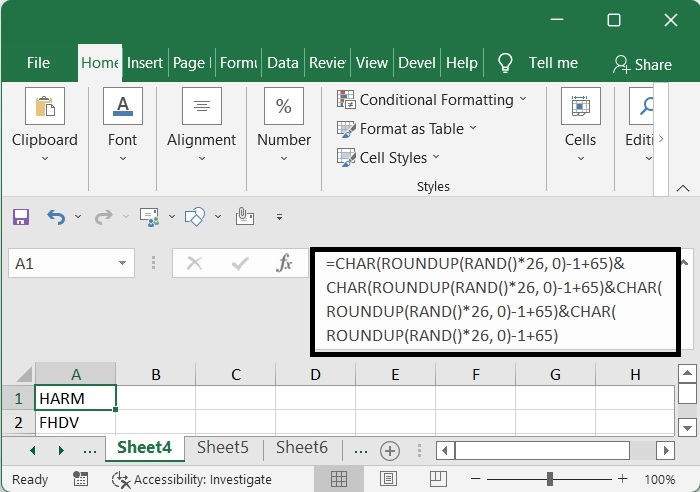Or

=CHAR(RANDBETWEEN(65, 90))&CHAR(RANDBETWEEN(65,90))&CHAR(RANDBETWEEN(65, 90))&CHAR(RANDBETWEEN(65, 90))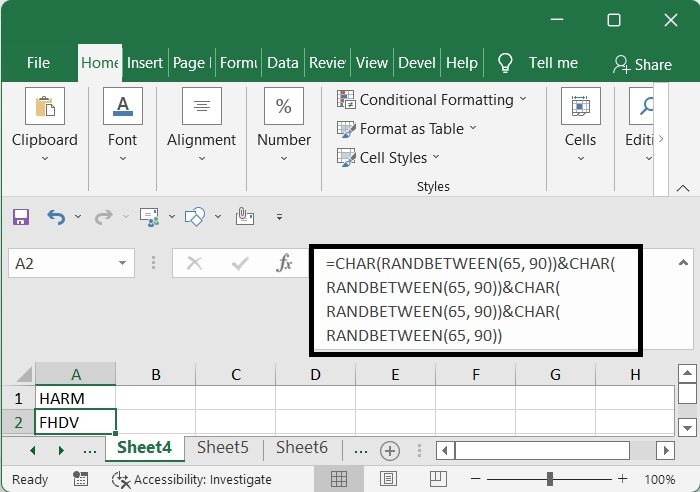### four random lowercase letters

By using the formula shown below, you should get four random lowercase letters.

=CHAR(ROUNDUP(RAND()*26, 0)-1+97)&CHAR(ROUNDUP(RAND()*26, 0)-1+97)&CHAR(ROUNDUP(RAND()*26, 0)-1+97)&CHAR(ROUNDUP(RAND()*26, 0)-1+97)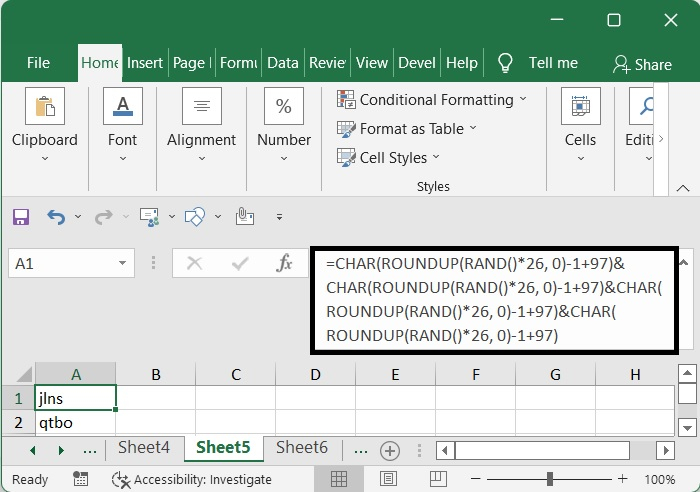Or

=CHAR(RANDBETWEEN(97, 122))&CHAR(RANDBETWEEN(97,122))&CHAR(RANDBETWEEN(97, 122))&CHAR(RANDBETWEEN(97, 122))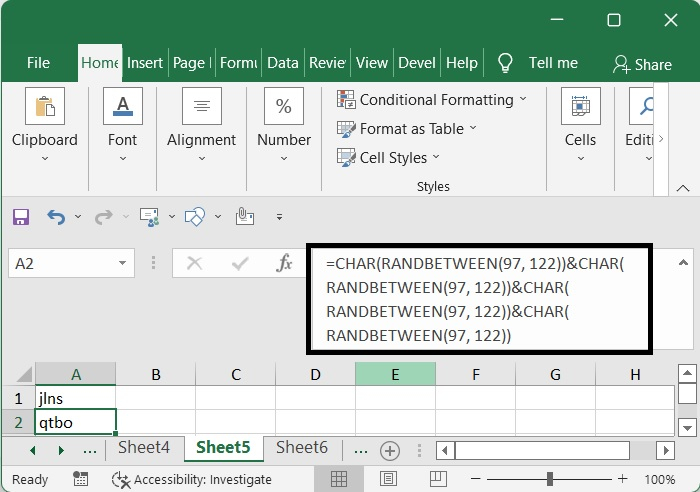The following formula will provide three random letters, which may be either uppercase or lowercase depending on your preference.

=IF(RAND()<0.5, CHAR(ROUNDUP(RAND()*26, 0)-1+65),CHAR(ROUNDUP(RAND()*26, 0)-1+97))&IF(RAND()<0.5,CHAR(ROUNDUP(RAND()*26, 0)-1+65), CHAR(ROUNDUP(RAND()*26, 0)-1+97))&IF(RAND()<0.5, CHAR(ROUNDUP(RAND()*26, 0)-1+65),
CHAR(ROUNDUP(RAND()*26, 0)-1+97))&IF(RAND()<0.5,CHAR(ROUNDUP(RAND()*26, 0)-1+65), CHAR(ROUNDUP(RAND()*26, 0)-1+97))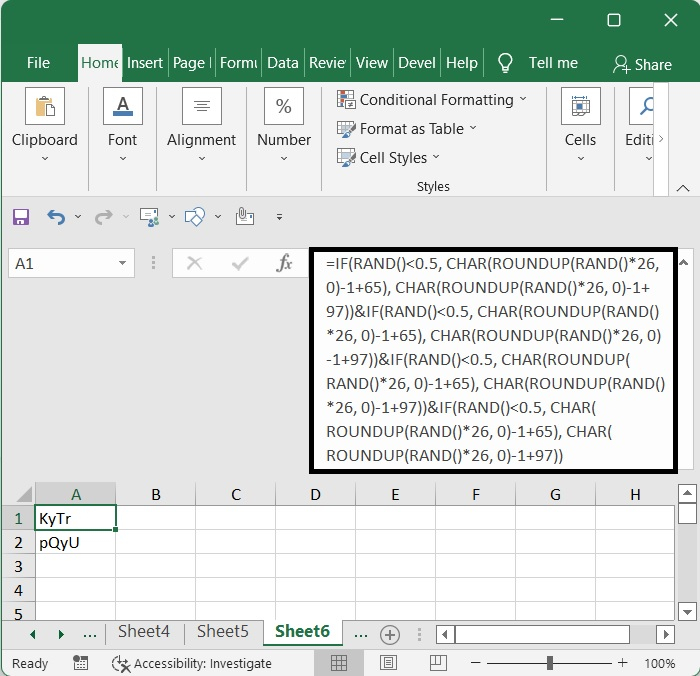Or

=IF(RAND()<0.5, CHAR(RANDBETWEEN(65, 90)),CHAR(RANDBETWEEN(97, 122)))&IF(RAND()<0.5,CHAR(RANDBETWEEN(65, 90)), CHAR(RANDBETWEEN(97,122)))&IF(RAND()<0.5, CHAR(RANDBETWEEN(65, 90)),8CHAR(RANDBETWEEN(97, 122)))&IF(RAND()<0.5,CHAR(RANDBETWEEN(65, 90)), CHAR(RANDBETWEEN(97, 122)))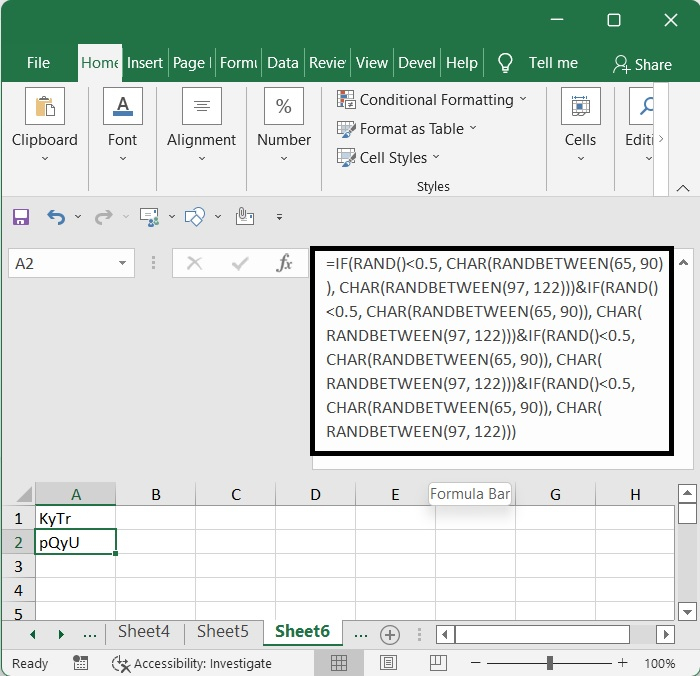## Random dates

Excel implements a method for representing dates that is known as the 1900-system. The oldest date that you are able to use is January 1, 1900, and it is denoted by the number 1. The current date, 2022-01-01, is 44562 days after the beginning of the century, 1900-01-01.

Now that we understand how dates are handled in Excel, let's imagine you want to generate a random number between 2022-01-01 and 2023- 01-01. 2022-01-01 is 44562 and 2023-01-01 is 44927. 44927- 44562 = 365 days.

=44562+ROUNDUP(RAND()*366,0)-1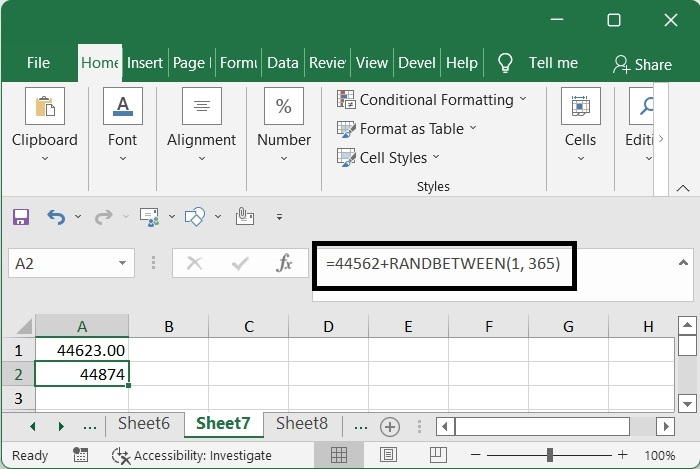Or

=44562+RANDBETWEEN(1, 365)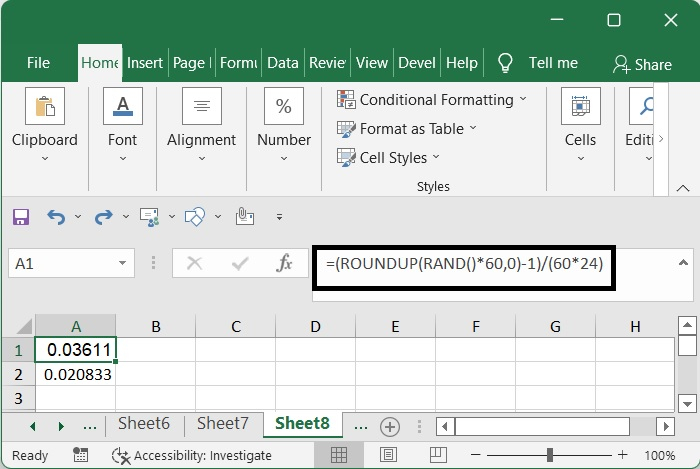## Random times

You may use the following formula to produce a random time between two specific times, such as generate time between 10 o'clock and 20 o'clock, if you desire to do so.

=TEXT(RAND()*(20-10)/24+11/24,"HH:MM:SS")


Enter the formula shown below into a cell that is otherwise empty, and then move the fill handle so that it is positioned over the range of cells into which you wish to enter the time.